Worksheet Question & Answers : Integers

# Worksheet Question & Answers : Integers - Mathematics (Maths) Class 7

Ques 1: Fill in the blanks:
(a) 30 ÷ ________ = 3
(b) – 89 ÷ ________ = 89
(c) ________ ÷ 1 = – 205
(d) ________ × (–12) = 120
(e) 24 + 12 ÷ 4 – 5 × 3 = ________
Ans:
(a) 10
Solution:
30/10 = 3
(b) –1

Solution:
– 89 ÷ -1 = 89
(c) –205

Solution:
When a number is divided by 1, the quotient is the number itself.
(d) –10

Solution:
let blank be X

X x(-12)=120

X=120/-12

X=-120/12

X=-10
= -10
(e)   12

Solution:
24+12 ÷ 4-5×3

=24+3-5×3

=24+3-15

=27-15

=12

Ques 2: Find the value of P, if p × (–9) = 135
Ans: P = –15

Solution:

• p× (-9) = 135 is given.
• We have to find the value of p.

We can simply find the value of p by solving the linear equation by transposing the terms.

p× (-9) = 135

Transposing -9 from LHS to RHS, we get

p  = 135/ (-9)

Simplifying 135/(-9), we get

p  = -15

∴ The value of p is (-15).

Hence, the value of p if  p× (-9) = 135 is (-15).

Ques 3: The additive inverse of zero (0) is ________.
Ans: 0
Solution: The additive inverse of a number is an integer that gives 0 as the sum when added.
E.g. - Let the required number be 3.
Then its additive inverse is -3.
since, -3 + 3 = 0

The additive inverse of 0 would be 0.
As, 0 + 0 = 0.

Ques 4: Match the properties of Integers:

 (a) Distributive law over addition (i) a + 0 = a = 0 + a (b) Associative law for multiplication (ii) a + b = b + a (c) Additive Identity (iii) (a × b) × c = a × (b × c) (d) Commutative law over addition (iv) a × 1 = 1 × a = a (e) Multiplicative Identity (v) a × (b + c) = a × b + a × c

Ans: (a) (v)
(b) (iii)
(c) (i)
(d) (ii)
(e) (iv)

Ques 5: Use the sign of >, < or = in the box to make the statement true.
(a) 25 – 40 + 10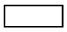25 – 40 – 10

1.   25 - 40 + 10

Ans: >

Sol:

We work from left to right:

25 - 40 + 10

25 - 40 = -15

So now we have -15 + 10

-15 + 10 = -5

Solving, 25 – 40 – 10

We work from left to right:

25 - 40 = -15

So now we have -15 - 10

= - 25

-5 > - 25

(b) (–9) + (–6)(–9) – (–6)

Ans: <

Sol:
(–9) + (–6) = -15
(–9) – (–6) = -3
-15< -3

(c) 35 + (–70) – (–35)(–24) – (15) + 39

Ans: =

Sol:
We work from left to right:

35 + (–70) = - 35
So,
- 35 - (-35) = 0

Solving, (–24) – (15) + 39
(–24) – (15)= -39
-39+ 39 = 0

Ques 6: Evaluate :
(a) (–1) × (–2) × (–3) × (–4) × (–5)
(b) 795 × (–25) + (–795) × 75
(c) (–59) × (–19) + 59
Ans:
(a) –120
Sol:

-1×(-2)×(-3)×(-4)×(-5)

= -2×(-12)×(-5)

= -24×(-5)

= -120

(b) –79500

Sol: 795×(-25)+(-795)×75

= -19875+(-59625)

= -19875-59625

= -79500

(c) 1180

Sol: -59×(-19)+59

= 1121+59

= 1180

Ques 7: An elevator descends into a mine shaft at the rate of 7m/min. If the descent starts from 5m above the ground level, how long will it take to reach – 205m?
Ans. 30 min.
Sol:

Total distance to be travelled = 5 m + 205 m = 210 m

Speed of descent = 7 m / min

Total Time = Distance / Speed

= 210 m / (7 m/min)

= 30 min

Ques 8: With the help of number line find how much greater is the number 3 than –3.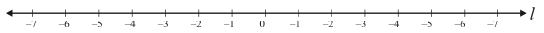Ans: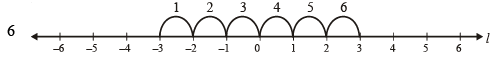Ques 9: The sum of two integers is –1500. One of the numbers is 599. Find the other number.
Ans: –2099
Sol: Let the number be X
the other number = 599
599 + X = 1500
X = 1500 - 599

X = 901

Ques 10: Write the answer in True/False :
(a) When 0 is divided by an integer, the quotient is zero.
(b) The product of an integer and 1 is an integer.
(c) When an integer is divided by itself, the quotient is 1.
Ans: (a) True
(b) True
(c) True

Ques 11: The product of two integers is –160. If one of them is 20, find the other.
Ans: –8
Sol:

Let the number be X

X * 20=-160

X =-160/20

X=-8

Ques 12: What integers should be multiplied by (–12) to becomes –300.
Ans: 25
Sol:
Let the integer be "a"
According to question,
(-12) × a = - 300
a = - 300 / - 12
a = 300 /12
a = 25
Required number = 25

Ques 13: Pallavi got a scholarship of Rs.1000 from her school. If she gives 550 for the help of flood relief, find the balance amount with her.
Ans: ₹ 450
Sol:
Total Amount = 1000
Rupees given for help = 550
Remaining balance of pallavi= 1000-550 = 450

Ques 14: A building has 21m in height. A monkey can climb 3m in one jump. In how many jumps, would the monkey reach at the top of the building?
Ans: 7
Sol: If the monkey could jump 3m once then he would have to jump 7 times to reach at top of the building as 7*3 = 21

Ques 16: Find solutions for the following problems:
a) Both numbers are less than 10.
The distance between the two numbers on the number line is 14.
b) Both numbers are greater than -15 and less than 5
One number is 6 greater than the other number.
c)The numbers are opposites.
The distance between the two numbers on the number line is 18.
d) One number is -35.
The distance between the two numbers on the number line is 20.
e) The first number is the opposite of -17.
The second number is less than the first number.
The distance between the two numbers on the number line is 9.

Sol: These questions can be easily solved using the number line.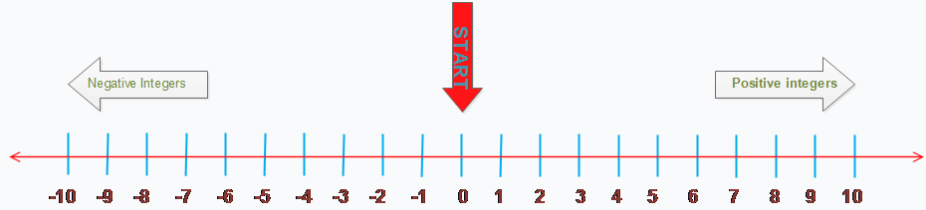a) There can be many solutions, one solution will be 7 and -7. They both are less 10 and difference is 14
Other solution could be -11 and 3.They both are less 10 and difference is 14
b) There can be many solutions, one solution will be -14 and -8.
Other solution could be -13 and -7.
c) This one will have unique solution. The number will be -9 and 9. Both are opposites and distance between them is 18
d) There can two solutions, one solution will be -55 and another one is -15
e) There can be one solution only. the solution is 17 and 8.

Ques 15: Fill in the blanks using different operations :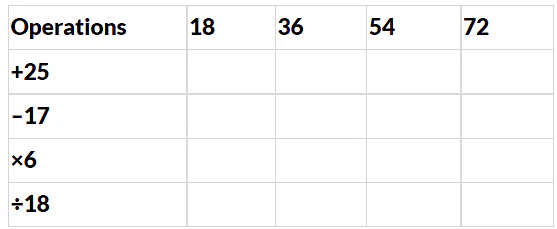Ans: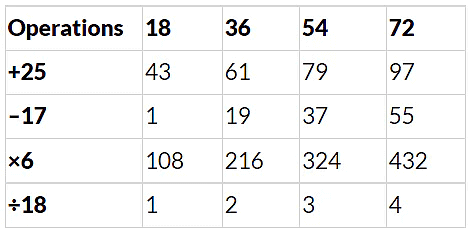The document Worksheet Question & Answers : Integers | Mathematics (Maths) Class 7 is a part of the Class 7 Course Mathematics (Maths) Class 7.
All you need of Class 7 at this link: Class 7

## Mathematics (Maths) Class 7

121 videos|351 docs|45 tests

## FAQs on Worksheet Question & Answers : Integers - Mathematics (Maths) Class 7

 1. What are integers?Ans. Integers are whole numbers that can be positive, negative, or zero. They do not have any fractional or decimal parts. Examples of integers are -3, 0, 5, and 10.
 2. How are integers different from natural numbers?Ans. Integers include both positive and negative numbers, whereas natural numbers only include positive whole numbers. Natural numbers start from 1, while integers can include negative numbers as well.
 3. How do you add integers?Ans. To add integers, you need to follow some rules. If you are adding two positive integers, simply add the numbers together. If you are adding two negative integers, add the numbers and then put a negative sign in front. If you are adding a positive and a negative integer, subtract the smaller number from the larger one, and use the sign of the larger number.
 4. Can integers be fractions or decimals?Ans. No, integers cannot be fractions or decimals. Integers are whole numbers that do not have any fractional or decimal parts. They are only represented by whole numbers.
 5. What is the difference between an integer and a rational number?Ans. Integers are a subset of rational numbers. While integers include whole numbers that can be positive, negative, or zero, rational numbers include integers as well as fractions or decimals. Rational numbers can be expressed as a ratio of two integers.

## Mathematics (Maths) Class 7

121 videos|351 docs|45 testsExplore Courses for Class 7 examSignup to see your scores go up within 7 days! Learn & Practice with 1000+ FREE Notes, Videos & Tests.
10M+ students study on EduRev
Track your progress, build streaks, highlight & save important lessons and more!
Related Searches

,

,

,

,

,

,

,

,

,

,

,

,

,

,

,

,

,

,

,

,

,

;